# Electronics and Communication Engineering - Signals and Systems

Exercise : Signals and Systems - Section 1
1.
What about the stability of system in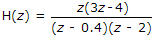system is stable
unstable
stable at 0.4
cant say
Explanation:

ROC include the unit circle hence it is stable.

2.
Which one most appropriate dynamic system?
y(n) + y(n - 1) + y(n + 1)
y(n) + y(n - 1)
y(n) = x(n)
y(n) + y(n - 1) + y(n + 3) = 0
Explanation:

Because present output of y(n) depend upon past y(n - 1) and future y(n + 1).

3.
An energy signal has G(f) = 10. Its energy density spectrum is
10
100
50
20
Explanation:

Energy density spectrum = |G(f)|2 = |10|2 = 100.

4.
Consider Pole zero diagram as shown, If two Poles are moved in opposite direction towards ω = p/2 and -p/2, the filter will be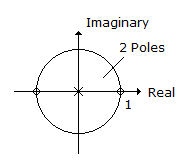change to high pass filter
change to Band Pass filter
remains same
change to LPF
Explanation: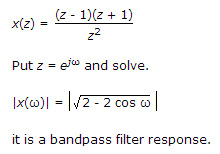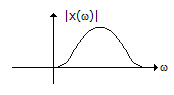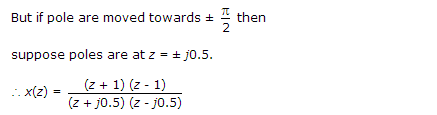But still we will get the same response, zero are at same position.

5.
In an ac circuit the fundamental component of current wave lags the corresponding voltage wave by 20°. The third harmonic component of current wave lags the corresponding voltage by an angle.
less than 20°
more than 20°
equal to 20°
equal to or more than 20°
For third harmonic θ = tan-1 =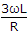.